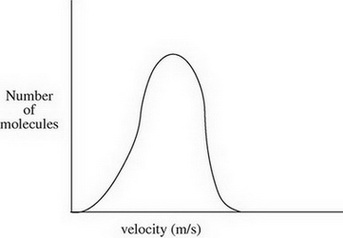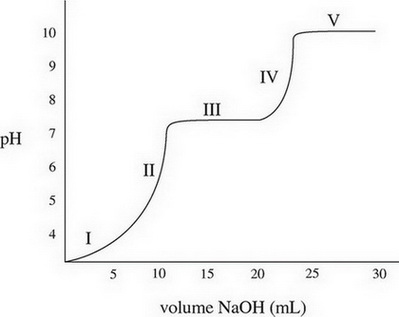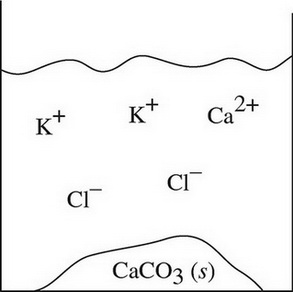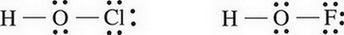# AP Chemistry Practice Test 2

### Test Information11 questions17 minutes

1.The diagram above shows the speed distribution of molecules in a gas held at 200 K. Which of the following representations would best represent the gas at a higher temperature? (Note: The original line is shown as a dashed line in the answer options.)

2. Nitrogen's electronegativity value is between those of phosphorus and oxygen. Which of the following correctly describes the relationship between the three values?

3. A sample of a compound known to consist of only carbon, hydrogen, and oxygen is found to have a total mass of 29.05 g. If the mass of the carbon is 18.02 g and the mass of the hydrogen is 3.03 g, what is the empirical formula of the compound?

`Questions 4-6 refer to the following information.A solution of carbonic acid, H2CO3, is titrated with sodium hydroxide, NaOH. The following graph is produced:`

4. In addition to OH-, what species are present in the solution during section III of the graph?

5. What is the magnitude of the first dissociation constant?

6. If the concentration of the sodium hydroxide is increased prior to repeating the titration, what effect, if any, would that have on the graph?

7. Two solutions of potassium carbonate and sodium chloride are mixed together, and the particulate representation below shows what is present after the reaction has gone to completion.Which of the two original solutions is the limiting reagent and why?

8. In which of the following circumstances is the value for Keq always greater than 1?

&DELTA;H &DELTA;S

9. The structure of two oxoacids is shown below:Which would be a stronger acid, and why?

10. During a chemical reaction, NO(g) gets reduced and no nitrogen-containing compound is oxidized. Which of the following is a possible product of this reaction?

11. Which of the following pairs of substances would make a good buffer solution?